0 49

# Data Mining and Warehousing - 3

Introduction to Data and Data Mining Data mining is the process of discovering patterns in large data sets involving met...

## Introduction to Data and Data Mining

Data mining is the process of discovering patterns in large data sets involving methods at the intersection of artificial intelligence, machine learning, statistics, and database systems.

The goal of data mining is to extract information from a data set and transform it into an understandable structure for further use.

### Data

Data refers to values of qualitative or quantitative variables about objects.

Types of data:

• Numerical - values represented by numbers like temperature, weight, etc.

• Categorical - values represented by categories like gender, colour, etc.

• Text - unstructured data like documents, articles, and social media posts.

• Image - photos, videos, diagrams, etc.

• Audio - recordings of sounds, voices, music, etc.

Data quality aspects:

• Accuracy - free from errors

• Completeness - contains all required information

• Consistency - uses the same definitions and formats

• Timeliness - up-to-date and recent

### Data mining

Data mining involves six major tasks:

1. Classification - predicting class labels

2. Regression - predicting continuous values

3. Clustering - grouping similar data points

4. Association rule learning - finding correlations

5. Anomaly detection - finding unusual patterns

6. Feature engineering - constructing features for modelling

## Data Types

Data can be categorized into different types based on its structure and format:### Structured data

Structured data has a predefined format and is organized in a tabular format like rows and columns. It is easy to query, analyze and store in databases.

Examples of structured data:

• Data in relational databases

• Data from sensors and RFID tags

Features of structured data:

• Organized in tables with fields, records and relationships

• Follows predefined data models

• Easy to query, search and aggregate

• Well-organized

• Easy to integrate with data mining and machine learning algorithms

### Unstructured data

Unstructured data does not have a predefined data model, format or schema. It includes text, images, audio and video.

Examples:

• Text documents, emails, social media posts

• Images, videos, audio recordings

• Sensor data, log files

Features:

• Raw format with no structure

• Difficult to query and analyze directly

• Contains valuable information not captured in structured data### Semi-structured data

Semi-structured data has some structure but not a predefined schema. It uses tags or other markers to denote fields, elements or attributes within the data.

Examples:

• XML and JSON documents

• Web pages

Features:

• Some structure defined by tags

• More flexible than structured data

• Easier to analyze than unstructured data

## Data Quality

For data to be useful for data mining and analysis, it needs to have high quality. The main aspects of data quality are:

### Accuracy

Accuracy refers to the correctness of the data and the degree to which it is free from errors.

Some ways to ensure accuracy:

• Data validation and checks

• Data cleaning to correct or remove incorrect values

• Using trusted and reliable sources

### Completeness

Completeness means the data contains all relevant information and attributes required for analysis.

Some ways to improve completeness:

• Identify missing data and fields

• Impute missing values using means, medians or other techniques

• Collect additional data to fill gaps

### Consistency

Consistency means the data uses the same definitions, formats, codes and units of measurement.

Some ways to ensure consistency:

• Define standards and rules for data collection

• Standardize data entry forms and templates

• Perform data integration and consolidation

### Timeliness

Timeliness refers to how recent or up-to-date the data is. Stale data loses its value.

Some ways to maintain timeliness:

• Set time limits for data refresh and update

• Collect data in real time through sensors, apps, etc.

• Archive old data that is no longer relevant

## Data Preprocessing

Data preprocessing refers to preparing raw data for data mining and machine learning algorithms. It involves cleaning, transforming and reducing data.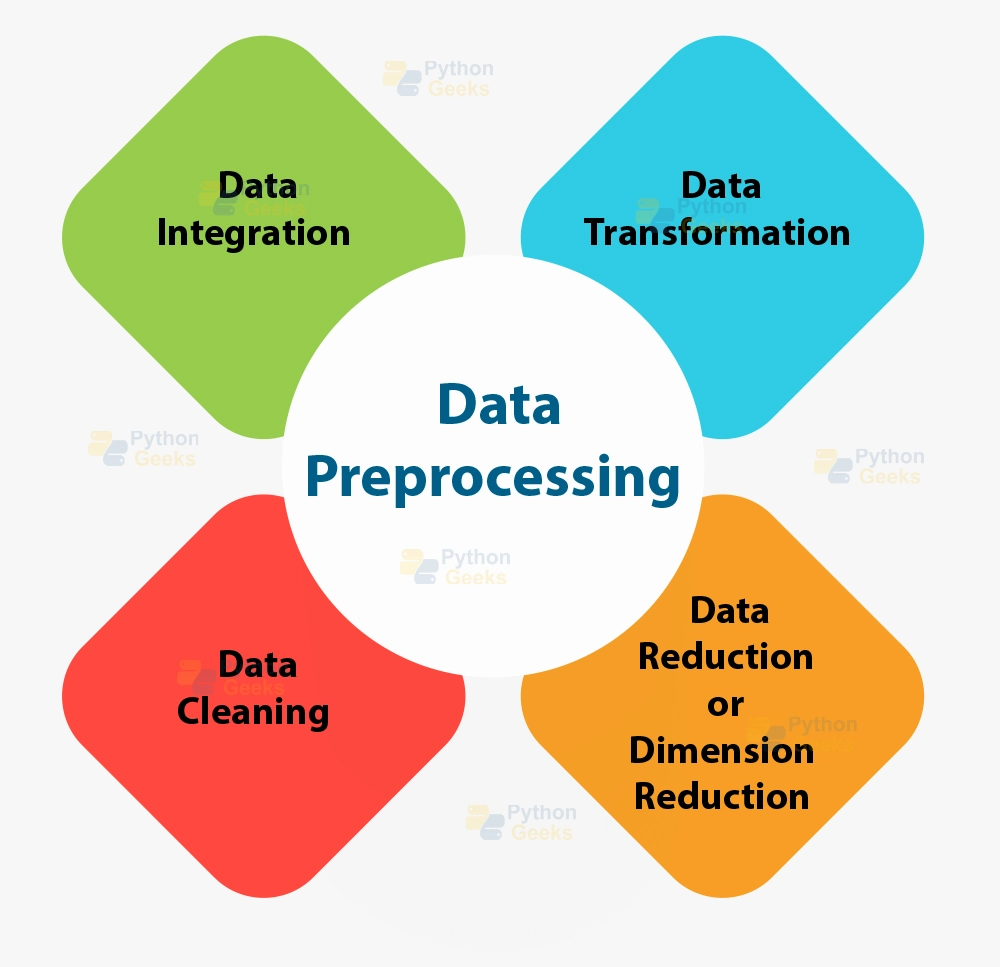### Data cleaning

Data cleaning involves:

• Removing noise and outliers

• Handling missing data

• Correcting inconsistencies

• Resolving duplicates

Techniques:

• Imputation for missing values

• Smoothing for noisy data

• Winsorization to cap outliers

Removal of noise and outliers:

Noise and outliers refer to data points that deviate from the main data distribution. They need to be identified and removed during data cleaning.

Noise:

Noise refers to data points that are erroneous or irrelevant. Common types of noise are:

• Typographical errors - Due to mistakes while entering data • Measurement errors - Due to faulty sensors or equipment • Subjective biases - Due to personal judgements while labelling data

Noise can be removed using:

• Thresholding - Removing points outside a certain range
• Clustering - Isolating noisy points into a separate cluster • Domain knowledge - Removing points that are known to be incorrect

Outliers:

Outliers are data points that are very different from the rest of the data. They can be:

• Extreme values - Data points that are unusually high or low

• Isolated points - Data points that are far from the main distribution

Outliers can be identified using:

• Standard deviation - Points more than 2-3 standard deviations away

• Interquartile range - Points above 1.5 times the interquartile range

• Distance-based - Points far from the mean or centroid of clusters

Once identified, outliers can be removed completely or capped to a threshold.

Some pros and cons of removing outliers:

• Pros - Reduce noise, improve model accuracy

• Cons - Can remove potentially useful information, distort data distribution

### Data integration

Data integration combines data from multiple sources by:

• Resolving schema differences

• Matching common attributes

• Eliminating duplicates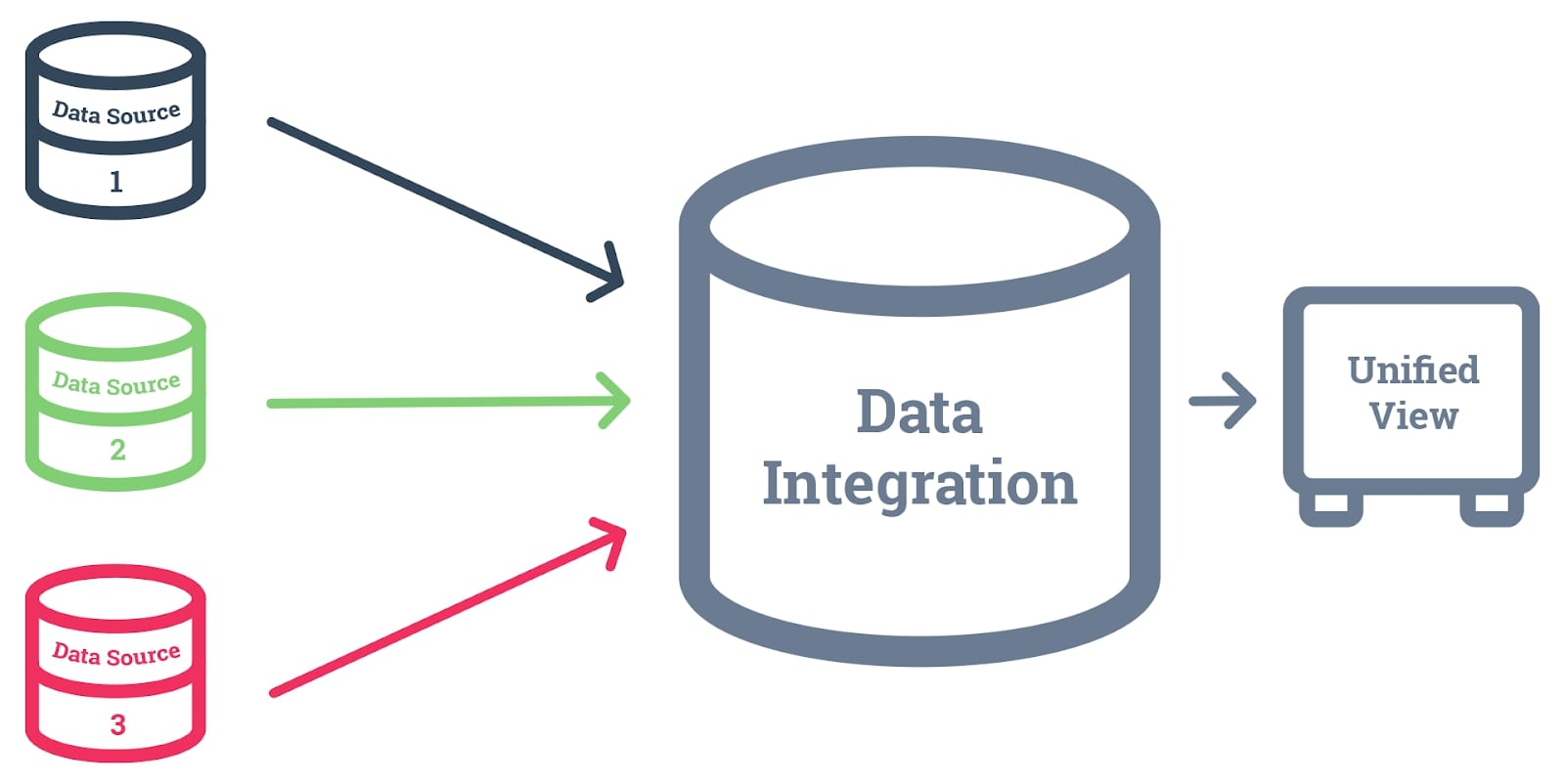Techniques:

• Schema mapping

• Data fusion

Merging multiple data sources:

Merging data from multiple sources is an important task in data integration during data preprocessing. It involves combining relevant information from different datasets.

The main steps in merging multiple data sources are:

1. Identify relevant sources: Select data sources that contain complementary information about the same entities.

2. Assess data quality: Check for inconsistencies, missing values, outliers and noise in each data source. Perform necessary data cleaning.

3. Match records: Identify records that refer to the same real-world entity across different data sources. This is done using record linkage techniques.

4. Resolve conflicts: Handle inconsistent information about the same entity from different sources. Various conflict resolution techniques can be used.

5. Merge data: Combine the relevant information from matched records into a single integrated record. Redundant information is removed.

6. Evaluate quality: Evaluate the quality of the merged dataset using metrics like completeness, consistency and accuracy.

Some techniques used for merging data are:

• Record linkage - Compare attributes like name, ID, etc. to match records
• Data fusion - Combine information from matched records
• Ensemble methods - Combine predictions from multiple models to get a better result

### Data transformation

Data transformation changes the format and scale of data:

• Normalization scales numeric attributes

• Discretization converts continuous attributes to discrete intervals

• Feature construction generates new attributes from existing ones

Techniques:

• Min-max normalization

• Z-score normalization

• Binning

Normalization:

Normalization is a process of transforming attributes in the dataset to make them fall within a small specified range, typically 0 to 1.

It is an important data transformation technique used during data preprocessing.

1. Min-max normalization

The original values are scaled to fall between a minimum and maximum value (typically 0 to 1):

x' = (x - min(x)) / (max(x) - min(x))

where x' is the normalized value and x is the original value.

Min-max normalization preserves all relationships in the original data.

1. Z-score normalization

The original values are scaled to have a mean of 0 and a standard deviation of 1:

x' = (x - mean(x)) / standard deviation(x)

Z-score normalization preserves relative differences between values and makes them comparable.

1. Decimal scaling

The original values are divided by a scaling factor (usually a power of 10) to reduce their range.

1. Other techniques:
• Tanh normalization

• Sigmoid normalization

The benefits of normalization include:

• Improved model accuracy

• Faster convergence for optimization algorithms

• Ease of comparison between attributes

### Data Reduction

Data reduction reduces data dimensionality by:

• Removing irrelevant or redundant attributes

• Grouping highly correlated attributes

Techniques:

• Principal component analysis (PCA)

• Linear discriminant analysis (LDA)

• Feature selection methods

Dimensionality reduction techniques:

Dimensionality reduction refers to the process of reducing the number of random variables under consideration by obtaining a set of principal variables that contain most of the information in the original high-dimensional data.

Common dimensionality reduction techniques are:

1. Feature selection

Involves selecting a subset of relevant features that contain the most information.

Techniques:

• Filter methods - Select features based on metrics like correlation, information gain, etc.

• Wrapper methods - Use machine learning to evaluate feature subsets.

• Embedded methods - Perform feature selection as part of model training.

1. Feature extraction

Involves transforming the features into a new set of uncorrelated variables called principal components.

Techniques:

• Principal Component Analysis (PCA) - Finds orthogonal principal components that capture the most variance in data.

• Linear Discriminant Analysis (LDA) - Finds components that best discriminate different classes.

• Multidimensional Scaling (MDS) - Preserves the pairwise distances between data points.

Other techniques:

• Autoencoders

• T-distributed Stochastic Neighbor Embedding (t-SNE)

## Similarity Measures

Similarity measures are used to quantify how similar or different two data objects are. They play an important role in data mining tasks like clustering, classification and recommendation systems.

There are two main types of similarity measures:

1. Distance measures

2. Correlation measures

### Distance measures

Distance measures quantify similarity as the inverse of distance between two data objects. Larger distances imply lower similarity and vice versa.

Common distance measures are:

1. Euclidean distance

It is the ordinary distance between two data points measured using the Pythagorean Theorem. Given two n-dimensional data points x and y, the Euclidean distance is calculated as:

d(x, y) = √∑(xi - yi)2

for i = 1 to n

Where n is the number of attributes.

1. Manhattan distance

It is the sum of the absolute differences of corresponding attributes. Given two n-dimensional data points x and y, the Manhattan distance is calculated as:

d(x, y) = ∑|xi - yi|

for i = 1 to n

Manhattan distance is faster to compute compared to Euclidean distance.

### Correlation measures

Correlation measures quantify similarity as the strength of association or correlation between two data objects. A higher positive correlation implies higher similarity.

Common correlation measures are:

1. Pearson correlation

It measures the linear correlation between two variables X and Y. The Pearson correlation coefficient r is calculated as:

r = ∑(xi - x̄)(yi - ȳ)/√∑(xi - x̄)2 ∑(yi - ȳ)2

1. Spearman correlation

It measures the monotonic correlation between two variables. It is calculated by first ranking the values of both variables and then calculating the Pearson correlation coefficient of the ranks.

## Summary Statistics

Summary statistics are used to summarize the main characteristics of a data set in a simple and concise form. They help provide an overview of the data distribution and identify any outliers or anomalies.

Common summary statistics used in data mining are:

### Mean, Median and Mode

1. Mean

The mean or average is the sum of all values divided by the number of values. It is calculated as:

Mean = (x1 + x2 + ... + xn) / n

The mean is highly influenced by outliers.

1. Median

The median is the middle value of an ordered list of values. It is the value that has an equal number of values above and below it.

The median is more robust to outliers compared to the mean.

1. Mode

The mode is the most frequent value in the data set. There may be multiple modes for a data set.

The mode provides information about the shape of the distribution.

### Variance and Standard Deviation

1. Variance

The variance is the average of the squared differences from the mean. It measures how spread out the values are. It is calculated as:

Variance = Σ(xi - Mean)2/n

where n is the number of values.

1. Standard Deviation

The standard deviation is the square root of the variance. It represents the typical distance between data points and the mean.

### Percentiles

Percentiles divide the data set into 100 equal parts. The nth percentile is the value below which n% of the observations fall.

For example, the 75th percentile is the value for which 75% of the observations have a lower value and 25% have a higher value.

Percentiles provide a simple summary of the data distribution.

## Data Distributions

Data distributions refer to the patterns in which data values are distributed in a data set. Understanding the distribution of data is important for data mining tasks like regression, clustering and outlier detection.

Common data distributions are:

### Normal distribution

Also known as Gaussian distribution.

It is a continuous probability distribution that is symmetric and bell-shaped. It is characterized by two parameters:

• Mean (μ): The average of the distribution

• Standard deviation (σ): How spread out the values are

The normal distribution is used widely as it approximates many natural phenomena.

It is represented by the probability density function:

f(x) = (1/(σ√(2π))) e^(-((x-μ)/2σ)^2)Where:

• μ is the mean or expected value

• σ is the standard deviation

• e is the base of the natural logarithm (approximately 2.71828)

### Binomial distribution

It describes the number of successes in a fixed number of independent yes/no experiments, each of which has a constant probability of success.

It is characterized by two parameters:

• n: Number of experiments

• p: Probability of success in a single experiment

The binomial distribution is used when modelling the number of successes in a sample.

The probability mass function is given by:

P(k) = (n!/(x!(n-x)!))p^x (1-p)^(n-x)Where:

• n is the number of trials

• x is the number of successes

• p is the probability of success in a single trial

### Poisson distribution

It describes the number of events occurring in a fixed interval of time and/or space when these events happen with a known average rate and independently of the time since the last event.

It is characterized by one parameter:

• λ: The expected number of occurrences during the interval.

The Poisson distribution is used when modelling the number of rare events occurring in an interval.

The probability mass function is given by:

P(k) = e^(-λ) λ^x /x!Where:

• λ is the mean number of occurrences during the interval

• x is the actual number of occurrences

The main data mining tasks are:

### Classification

Classification is the task of assigning data points to predefined categories or classes.

It involves building a model based on labelled training data where the class labels are known. The model can then be used to predict the class labels of new, unlabeled data.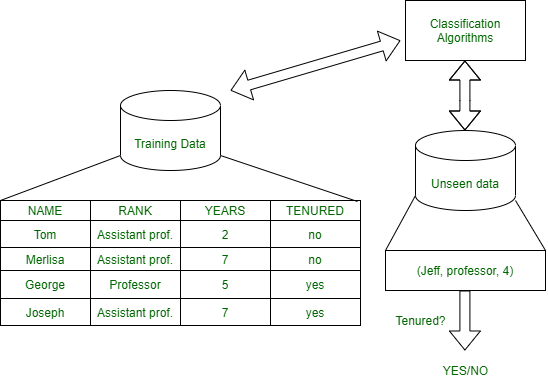Examples:

• Predicting if an email is spam or not spam

• Detecting fraudulent credit card transactions

Algorithms: Decision trees, Naive Bayes, KNN, SVM, Neural networks, etc.

### Clustering

Clustering is the task of grouping unlabeled data points into meaningful clusters such that data points within a cluster are similar and different clusters are dissimilar.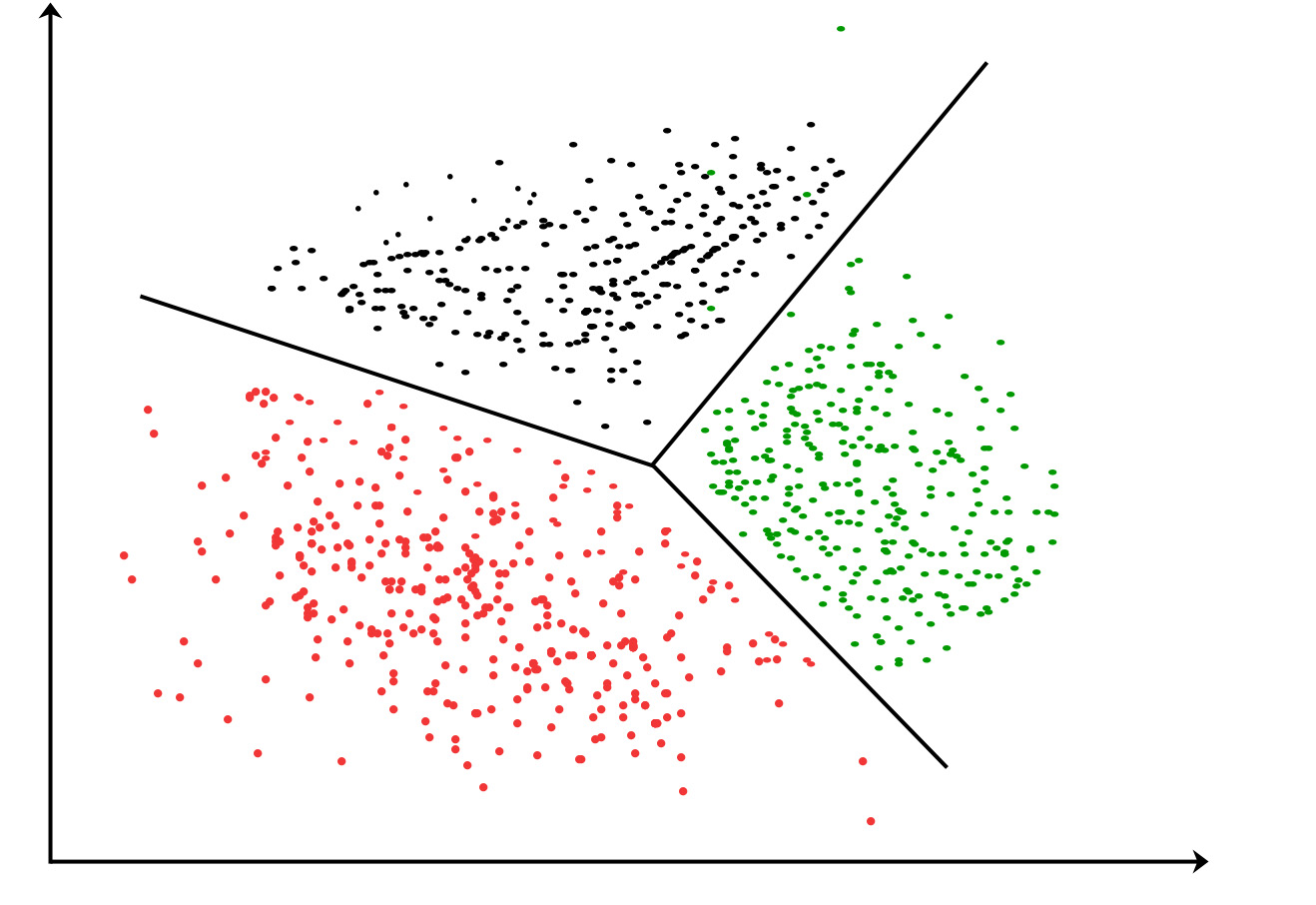The number of clusters is not predefined. The algorithm determines the clusters automatically.

Examples:

• Grouping customers based on purchasing behaviour

• Categorizing web pages

Algorithms: K-means, Hierarchical clustering, DBSCAN, etc.

### Association Rule Mining

It finds interesting relationships or associations among variables in a large data set.

It analyzes the frequency of occurrence of items together and derives rules that specify which items tend to co-occur.Examples:

• People who view product A also tend to view product B.

Algorithms: Apriori, Eclat

### Anomaly Detection

It identifies rare items, events or observations that raise suspicions by deviating from normal behaviour.It involves modelling normal behaviour and then looking for significant deviations from that behaviour.

Examples:

• Detecting credit card fraud

• Identifying system performance issues

Algorithms: Density-based methods, Clustering-based methods, Statistical methods

## Data Mining vs KDD

### Data Mining

• Data mining refers specifically to the algorithmic processes involved in extracting patterns from data.

• It involves applying machine learning, statistical and visualization techniques to uncover hidden patterns.

• Data mining focuses on the algorithms and techniques for discovering patterns.

### Knowledge Discovery in Databases (KDD)

• KDD refers to the overall process of discovering useful knowledge from data.

• It includes data cleaning, data integration, data selection, data mining and interpretation of the discovered patterns.

• KDD involves more data preparation and preprocessing steps before applying data mining algorithms.

Data MiningKnowledge Discovery in Databases
Focuses on the algorithmic part of pattern extraction from dataFocuses on the entire process of discovering useful knowledge from data
Involves less human interventionInvolves human decisions at various stages
Works with ready-to-mine dataIncludes data preparation and preprocessing steps
Covers pattern extractionCovers data understanding, cleansing and integration
Discovery of patternsEvaluation and interpretation of patterns to create knowledge
Uses machine learning and statistical techniquesUses machine learning, statistics, visualization and database techniques

## Issues in Data Mining

Data mining promises many benefits, but there are also some issues and challenges that need to be addressed for effective data mining.

1. ### Data Quality Issues

Data quality issues arise due to incomplete, noisy or irrelevant data. This affects mining accuracy and results.

Incomplete or Missing Data

• Data may be missing values for certain attributes due to errors in data collection or storage.

• This can lead to inaccurate or incomplete patterns during mining since the full picture is not available.

• Techniques like imputation can be used to fill in missing values but introduce some inaccuracy.

Noisy or Inconsistent Data

• Real-world data often contains errors, outliers and inconsistencies that reduce its quality.

• Noisy data makes it difficult for mining algorithms to identify true patterns and relationships.

• Data cleaning and filtering techniques can improve data quality but are not perfect.

Irrelevant Attributes

• Datasets often contain attributes that are not relevant to the mining task.

• Irrelevant attributes introduce "noise" that reduces the effectiveness of mining algorithms.

• Feature selection techniques can identify and remove irrelevant attributes but require domain knowledge.

1. ### Ethical Issues

Data mining raises some ethical concerns regarding privacy, security and proper use of results.

Privacy and Security

• Data mining can reveal sensitive information about individuals present in the data.

• Proper security measures and access controls are needed to protect personal data during and after mining.

Misuse of Data

• Data mining results could potentially be misused for fraudulent purposes such as target marketing scams.

• There are also concerns about possible discrimination based on patterns found in the data.

Lack of Transparency

• The complex algorithms used in data mining are often seen as a "black box" lacking transparency.

• It is difficult for users to verify and audit the mining process and results.

1. ### Scalability Issues

Large and complex datasets pose scalability challenges for data mining algorithms.

High Dimensionality

• Data with many attributes (high dimensionality) can reduce mining performance.

• Mining algorithms have difficulty identifying meaningful patterns in high-dimensional data.

• Dimensionality reduction techniques can help address the "curse of dimensionality".

Large Databases

• Mining very large databases can be computationally intensive and time-consuming.

• Traditional algorithms may need to be scaled using techniques like sampling, clustering and grid computing.

• ### Evaluation Issues

Issues related to correctly evaluating the quality of patterns found during mining.

Overfitting

• Models that are too tuned to the training data may not generalize well to new, unseen data.

• This is known as overfitting and results in poor performance on new data.

Subjectivity of Patterns

• The patterns found depend on the selected mining algorithm and its parameters.

• Different algorithms may discover different patterns in the same data.

Interpreting Results

• Users may find it difficult to understand and correctly interpret the discovered patterns.

• This is due to the complexity of patterns and lack of context about the mining process.

## Introduction to Fuzzy Sets and Fuzzy Logic

### Fuzzy sets

• Fuzzy sets are a generalization of ordinary sets where elements can have partial membership.

• In ordinary sets, elements either belong (membership = 1) or do not belong (membership = 0).

• In fuzzy sets, elements have a membership value between 0 and 1 indicating the degree of belonging.

• Fuzzy sets are useful for representing imprecise, vague or uncertain concepts.Example

Consider the set of "tall people". In an ordinary set, a person either belongs (is tall) or does not belong (is not tall).

In a fuzzy set, we can represent degrees of tallness using a membership value between 0 and 1:

• A person of height 180 cm may have a tallness membership value of 0.9

• A person of height 160 cm may have a tallness membership value of 0.3

• A person of height 140 cm may have a tallness membership value of 0.1

### Membership functions

• Membership functions are used to represent the degree of membership of elements in a fuzzy set.

• Common types of membership functions are:

• Triangular

• Trapezoidal

• Gaussian (Bell-shaped)

• Sigmoidal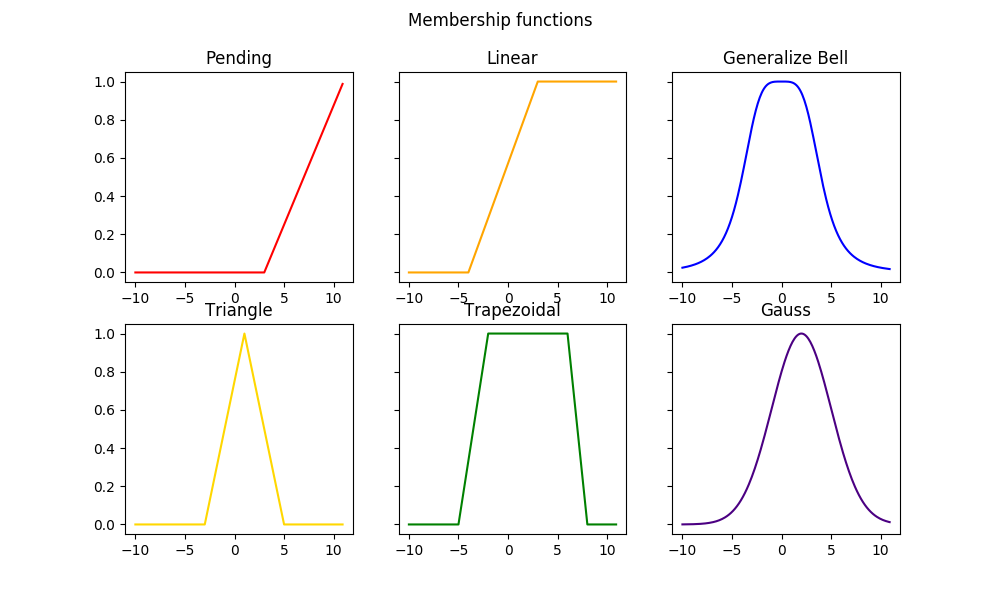• The x-axis represents the input variable and the y-axis represents the membership value from 0 to 1.

• Membership functions are used to map crisp input values to fuzzy membership values.

### Fuzzy Logic Operations

• Basic fuzzy logic operations are used to combine fuzzy sets.

• Common operations are:

• Union (OR): Takes the maximum membership value.

• Intersection (AND): Takes the minimum membership value.

• Complement (NOT): Subtracts the membership value from 1.

• These operations allow us to perform fuzzy inference and reasoning.Subscribe 🔥